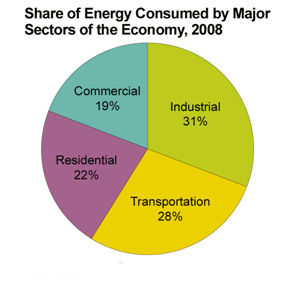Wiki
Home » Blog » Wiki » What is true power and apparent power?

# What is true power and apparent power?

KW is true power and KVA is apparent power. In per unit calculations the more predominantly used base, which I consider standard is the KVA, the apparent power because the magnitude of the real power (KW) is variable / dependent on a changing parameter of the cos of the angle of displacement (power factor) between the voltage and current. Also significant consideration is that the rating of transformers are based in KVA, the short circuit magnitudes are expressed in KVA or MVA, and the short circuit duty of equipment are also expressed in MVA (and thousands of amperes, KA ).

In per unit analysis, the base values are always base voltage in kV and base power in kVA or
MVA. Base impedance is derived by the formula (base kV)^2/(base MVA).

The base values for the per unit system are inter-related. The major objective of the per unit system is to try to create a one-line diagram of the system that has no transformers (transformer ratios) or, at least, minimize their number. To achieve that objective, the base values are selected in a very specific way:
a) we pick a common base for power (I'll come back to this point, if it should be MVA or MW);
b) then we pick base values for the voltages following the transformer ratios. Say you have a generator with nominal voltage 13.8 kV and a step-up transformer rated 13.8/138 kV. The "easiest" choice is to pick 13.8 kV as the base voltage for the LV side of the transformer and 138 kV as the base voltage for the HV side of the transformer.
c) once you have selected a base value for power and a base value for voltage, the base values for current and impedance are defined (calculated). You do not have a degree of freedom in picking base values for current and impedance.

Typically, we calculate the base value for current as Sbase / ( sqrt(3) Vbase ), right? If you are using that expression for the base value for currents, you are implicitly saying that Sbase is a three-phase apparent power (MVA) and Vbase is a line-to-line voltage. Same thing for the expression for base impedance given above. So, perhaps you could choose a kW or MW base value. But then you have a problem: how to calculate base currents and base impedances? If you use the expressions above for base current and base impedance, you are implicitly saying that the number you picked for base power (even if you picked a number you think is a MW) is actually the base value for apparent power, it is kVA or MVA. If you insist on being different and really using kW or MW as the base for power, you have to come up with new (adjusted) expressions for calculating base current and base impedance.

And, surprise!, you will find out that you need to define a "base power factor" to do so. In other words, you will be forced back into defining a base apparent power. So, no, you cannot (easily) use a kW/MW base. For example, a 100 MVA generator, rated 0.80 power factor (80 MW). You could pick 80 as the base power (instead of 100). But if you are using the expressions above for base current and base impedance, you are actually saying that the base apparent power is 80 MVA (not a base active power of 80 MW).

Calculate (3 + 3) =

You may also like:

As per Torque/Slip characteristic for AC Motor, the value of the Max. Torque can be developed is constant while the Starting Torque occurs @ S=.1, (T proportional to r2 and S also proportional to r2 where r2 ...
When the transition between wye and delta takes place, be very sure that that the mechanical interlocks on the contractors are properly adjusted. If one doesn't completely open before the other closes, you ...
The potential for variable frequency drive (VFD) energy saving from slowing down the load depend on the characteristics of the load being driven. There are three main types of load: variable torque, constant ...
We get at the poles of the system by looking at the characteristic equation, 1+T(s). Unfortunately, we don't have the math available (except in classroom exercises) we have an empirical system that may or may ...
In a balanced 3-phase system with pure sine waves, the neutral current is zero, ideally. If there is phase imbalance, it shows up in the neutral, so check for imbalance. The other major cause of high neutral ...Gozuk Blog: all about electric motor control & drives industries development in energy saving applications.

Featured

Like pumps, fans consume significant electrical energy while serving several applications. In many plants, the VFDs (variable ...A frequency inverter controls AC motor speed. The frequency inverter converts the fixed supply frequency (60 Hz) to a ... Motor starter (also known as soft starter, motor soft starter) is a electronic device integrates soft start, soft stop, ... Soft starter allows the output voltage decreases gradually to achieve soft stop, in order to protect the equipment. Such as the ... Soft Starter reduces electric motor starting current to 2-4 times during motor start up, reduces the impact to power grid during ...

In Discussion